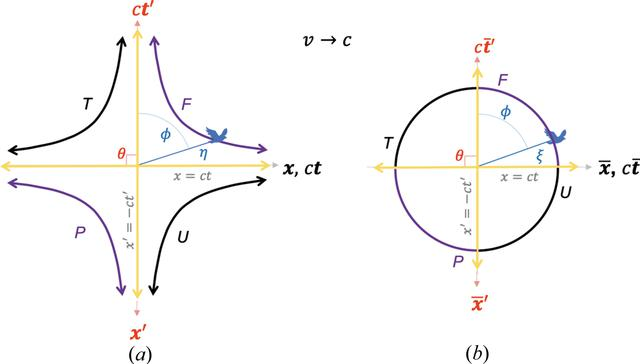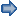disable zoom     view article Figure 8 A special case of the blended and the RBS coordinates, where. (a) A plot of the blended coordinates given in equations (7a)and (8). (b) RBS coordinates, given in equation (11b), transform (a) into a circle of radius, where the four hyperbola branches in (a) become four arc segments of the circle. Purple (black) hyperbola branches and arc segments represent directions from the origin where time-like (space-like) events occur for a fixed. Blended and RBS light lines are shown by yellow lines. See the Mathematica script in the Mathematica notebook in the supporting information to generate these plots.FOUNDATIONSADVANCES
ISSN: 2053-2733
Volume 77| Part 4| July 2021| Pages 242-256